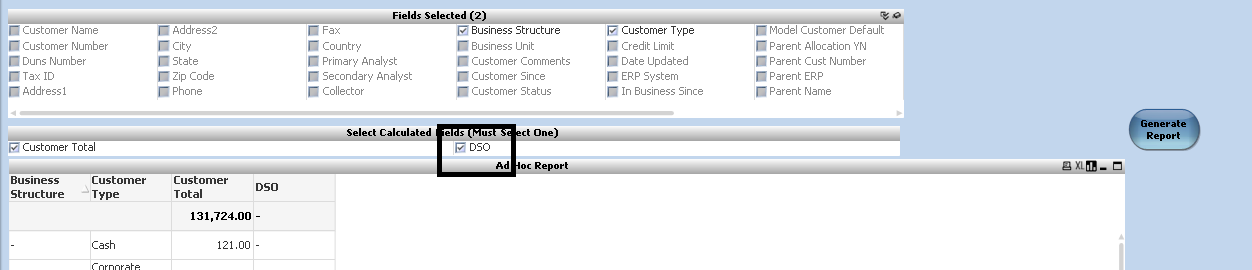# New to QlikView

Discussion board where members can get started with QlikView.

Announcements
Modernize Your QlikView Deployment webinar, Nov. 3rd. REGISTER
cancel
Showing results for
Did you mean:
HighlightedContributor

## How to add an expression using a date variable in the script

Hi Everyone, I'm new to QlikView and am having a little trouble modifying a report built by the previous person. We have an ad hoc report that allows the user to pick the dimension fields and the calculated fields displayed in the straight table. The calculated fields are defined in the script with an Expression table. What I trying to do is add a new calculated field that calculates DSO based on selections (start and end date) made in an input box. My problem is finding the correct syntax for the expression using the date variables (if that is even possible).

The user will select the desired dimensions and calculated fields the generate the report.The expression is defined in the script using a Expression table:The problem is when I add this expression the check box for DSO disappears from the List Box.

Round(Sum(if({<Ending Date = ('\$(vEndDateRange)', EndingAmount,0))/Sum(if({Invoice Date > ('\$(vStateDateRange)' and {Invoice      Date} < ('\$(vEndDateRange)', {Invoice Amount}, 0)) * (('\$(vEndDateRange)'-('\$(vStateDateRange)')

Does anyone have an idea how I can make this work?

2 Replies
HighlightedCreator III

hi

Create one variable for that expression like below

vExpression=MaxString(%Expression)

and use in table expression like below

\$(vExpression)

Also find attached qvw file.

Regards

Ahmar

HighlightedCreator III

or second option for you is use direct expression like below into your chart expression

Expression =\$(=maxstring(%Expression))